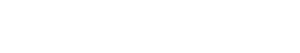# Prediction Interval: Simple Definition, Examples

Regression Analysis > Prediction Interval

## What is a Prediction Interval?Regression analysis is used to predict future trends.
A prediction interval is a type of confidence interval (CI) used with predictions in regression analysis; it is a range of values that predicts the value of a new observation, based on your existing model.

Prediction and confidence intervals are often confused with each other. However, they are not quite the same thing.

• A confidence interval is a range of values associated with a population parameter. For example, the mean of a population.
• A prediction interval is where you expect a future value to fall.

## The Uncertainties with Intervals

Just like most things in statistics, it doesn’t mean that you can predict with certainty where one single value will fall.

Confidence intervals are always associated with a confidence level, representing a degree of uncertainty (data is random, and so results from statistical analysis are never 100% certain).
For example, you might say that the mean life of a battery (at a 95% confidence level) is 100 to 110 hours. This tells you that a battery will fall into the range of 100 to 110 hours 95% of the time.

Similarly, the prediction interval tells you where a value will fall in the future, given enough samples, a certain percentage of the time. A 95% prediction interval of 100 to 110 hours for the mean life of a battery tells you that future batteries produced will fall into that range 95% of the time. There is a 5% chance that a battery will not fall into this interval.

## When to Use It

It’s very common to use the confidence interval in place of the prediction interval, especially in econometrics. However, you should use a prediction interval instead of a confidence level if you want accurate results. Let’s say you calculate a confidence interval for the mean daily expenditure of your business and find it’s between \$5,000 and \$6,000. That tells you where the mean probably lies. If you use that CI to make a prediction interval, you will have a much narrower interval. For example, the prediction interval might be \$2,500 to \$7,500 at the same confidence level. If you do use the confidence interval, it’s highly likely that interval will have more error, meaning that values will fall outside that interval more often than you predict.

Be careful when interpreting prediction intervals and coefficients if you transform the response variable: the slope will mean something different and any predictions and confidence/prediction intervals will be for the transformed response (Morgan, 2014).

## How to Find a Prediction Interval

By hand, the formula is:You probably won’t want to use the formula though, as most statistical software will include the prediction interval in output for regression. Look for it next to the confidence interval in the output as 95% PI or similar wording.

References:
Guang-Hwa “Andy” Chang. Linear Regression in SPSS. Retrieved July 3, 2017 from: http://gchang.people.ysu.edu/SPSSE/SPSS_lab2Regression.pdf
Morgan, K. (2014). Simple Linear Regression.

CITE THIS AS:
Stephanie Glen. "Prediction Interval: Simple Definition, Examples" From StatisticsHowTo.com: Elementary Statistics for the rest of us! https://www.statisticshowto.com/prediction-interval/
---------------------------------------------------------------------------Need help with a homework or test question? With Chegg Study, you can get step-by-step solutions to your questions from an expert in the field. Your first 30 minutes with a Chegg tutor is free!